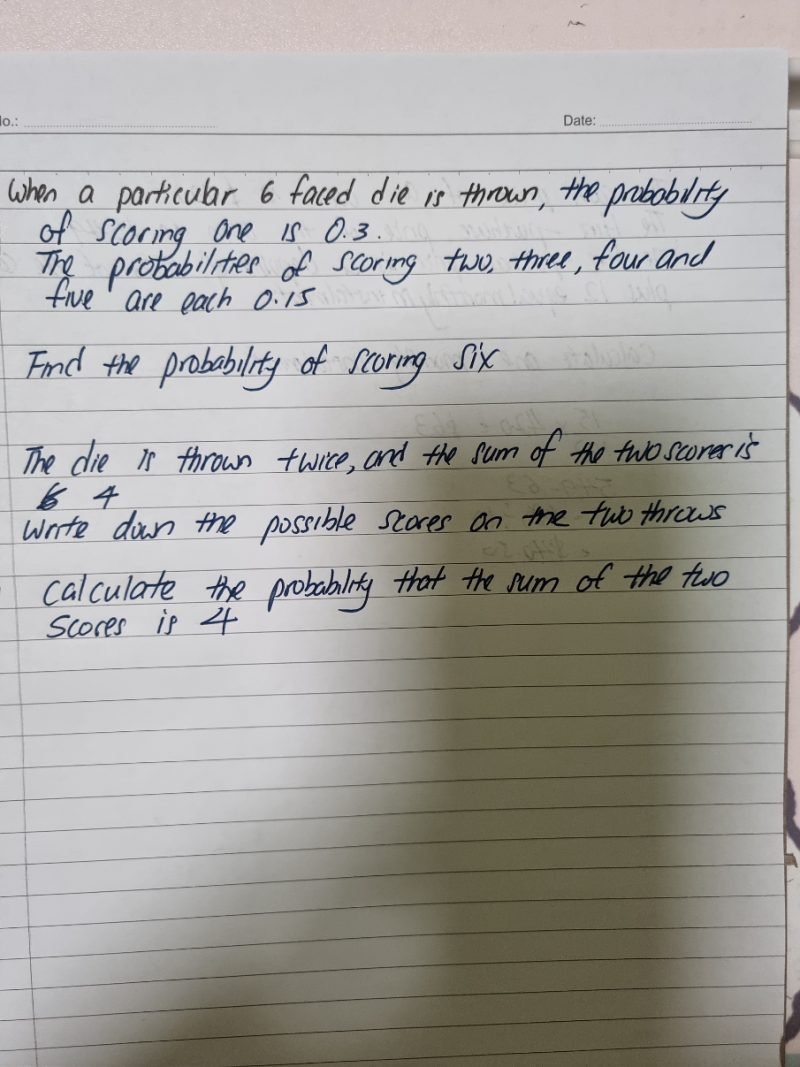# QuestionThank you

Prob (1) = 0.3

Prob (2) = Prob (3) = Prob(4) = Prob (5) = 0.15

Therefore, Prob (6) = 1 – 0.3 – 4(0.15) = 1-0.3 -0.6 = 0.1

The possible scores to get the sum of 4 are:

First try    Second try

1                  3

3                  1

2                  2

Probability of getting 1 on first try and 3 on second try = 0.3 x 0.15 = 0.045

Probability of getting 3 on first try and 2 on second try = 0.15 x 0.3 = 0.045

Probability of getting 2 on first try and 2 on second try = 0.15 x 0.15 = 0.0225

Therefore probability of getting sum of 2 scores as 4 = 0.045 + 0.045 + 0.0225 =  0.1125

0 Replies 1 Like